# Class 8 Maths NCERT Solutions for Chapter – 7 Cubes and Cube Roots Ex – 7.2

## Cubes and Cube Roots

Question 1.
Find the cube root of each of the following numbers by prime factorisation method :
(i)
64
(ii) 512
(iii) 10648
(iv) 27000
(v) 15625
(vi) 13824
(vii) 110592
(viii) 46656
(ix) 175616
(x) 91125

Solution:
(i) Resolving 64 into prime factors, we get
64 = 2 x 2 x 2 x 2 x 2 x 2
∴$\sqrt [ 3 ]{ 64 } =\left( 2\times 2 \right) =4$(ii) Resolving 512 into prime factors, we get
512 = 2 x 2 x 2 x 2 x 2 x 2 x 2 x 2 x 2
∴$\sqrt [ 3 ]{ 512 } =\left( 2\times 2\times 2 \right) =8$(iii) Resolving 10648 into prime factors, we get
10648 = 2 x 2 x 2 x 11 x 11 x 11
∴$\sqrt [ 3 ]{ 10648 } =\left( 2\times 11 \right) =22$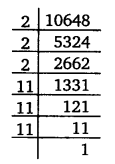(iv) Resolving 27000 into prime factors, we get
27000 = 1000 x 27
= 10 x 10 x 10 x 3 x 3 x 3 = 2 x 5 x 2 x 5 x 2 x 5 x 3 x 3 x 3
= 2 x 2 x 2 x 3 x 3 x 3 x 5 x 5 x 5
∴$\sqrt [ 3 ]{ 27000 } =2\times 3\times 5=30$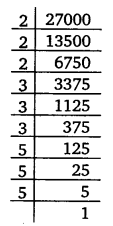(v) Resolving 15625 into prime factors, we get
15625 = 5 x 5 x 5 x 5 x 5 x5
∴$\sqrt [ 3 ]{ 15625 } =5\times 5=25$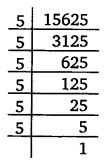(vi) Resolving 13824 into prime factors, we get
13824 = 2 x 2 x 2 x 2 x 2 x 2 x 2 x 2 x 2 x 3 x 3 x 3
∴$\sqrt [ 3 ]{ 13824 } =\left( 2\times 2\times 2\times 3 \right) =24$(vii) Resolving 110592 in prime factors, we get
110592 = 2 x 2 x 2 x 2 x 2 x 2 x 2 x 2 x 2 x 2 x 2 x 2 x 3 x 3 x 3
∴$\sqrt [ 3 ]{ 110592 } =\left( 2\times 2\times 2\times 2\times 3 \right) =48$(viii) Resolving 46656 in prime factors, we get
46656 = 2 x 2 x 2 x 2 x 2 x 2 x 3 x 3 x 3 x 3 x 3 x 3
∴$\sqrt [ 3 ]{ 46656 } =\left( 2\times 2\times 3\times 3 \right) =36$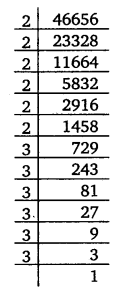(ix) Resolving 175616 in prime factors, we get
175616 = 2 x 2 x 2 x 2 x 2 x 2 x 2 x 2 x 2 x 7 x 7 x 7 = (2 x 2 x 2 x 7)
= 56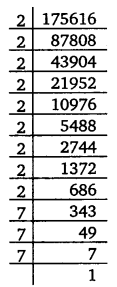(x) Resolving 91125 in prime factors, we get
91125 = 3 x 3 x 3 x 3 x 3 x 3 x 5 x 5 x 5
∴$\sqrt [ 3 ]{ 91125 } =\left( 3\times 3\times 5 \right) =45$Question 2.
State true or false :
(i) Cube of any odd number is even.
(ii) A perfect cube does not end with two zeros.
(iii) If square of a number ends with 5, then its cube ends with 25.
(iv) There is no perfect cube which ends with 8.
(v) The cube of a two digit number may be a three digit number.
(vi) The cube of a two digit number may have seven or more digits.
(vii) The cube of a single digit number may be a single digit number.

Solution:
(i) False
(ii) True
(iii) False, as 152 = 225 and 153 = 3375
(iv) False, as 8 = 23, 1728 =123, etc.
(v) False
(vi) False, as 103 = 1000, 993 = 970299
(vii) True, as 13 = 1, 23 =8.

Question 3.
You are told that 1,331 is a perfect cube. Can you guess without factorisation what is its cube root? Similarly, guess the cube roots of 4913, 12167, 32768.

Solution:
For 1331 :
Units digit of the cube root of 1331 is 1 as unit’s digit of the cube root of numbers ending in 1 is 1 . After striking three digits from the right of 1331, we get the number 1. Since 13 = 1, so the ten’s digit of the cube root of given number is 1.
∴$\sqrt [ 3 ]{ 1331 } =11$

For 4913 :
Units digit of the cube root of 4913 is 7 as unit’s digit of cube root of numbers ending in 3 is 7. After striking three digits from the right of 4913, we get the number 4. As 13 =1 and 23 =8, so 13 < 4 < 23. Therefore, the ten’s digit of cube root of 4913 is 1.
∴$\sqrt [ 3 ]{ 4913 } =17$

For 12167 :
Unit’s digit of the cube root of 12167 is 3 as unit’s digit of cube root of numbers ending in 7 is 3. After striking three digits from the right of 12167, we get the number 12. As 23 =8 and 33 =27, so 23 < 12 < 33. So, the ten’s digit of the cube root of 12167 is 2.
∴$\sqrt [ 3 ]{ 12167 } =23$

For 32768 :
Unit’s digit of the cube root of 32768 is 2 as unit’s digit of cube root of numbers ending in 8 is 2. After striking three digits from the right of 32768, we get the number 32. As 33 =27 and 43 = 64, so 33 < 32< 43. So, the ten’s digit of the cube root of 32768 is 3.
∴$\sqrt [ 3 ]{ 32768 } =32$

### Take Your IIT JEE Coaching to Next Level with Examtube

• Mentoring & Teaching by IITians
• Regular Testing & Analysis
• Preparation for Various Engineering Entrance Exams
• Support for School/Board Exams